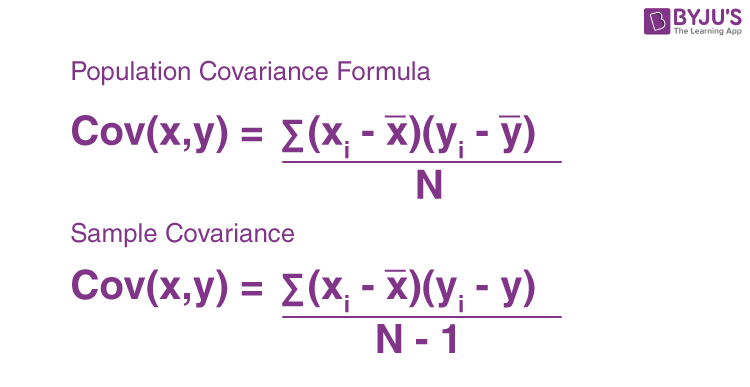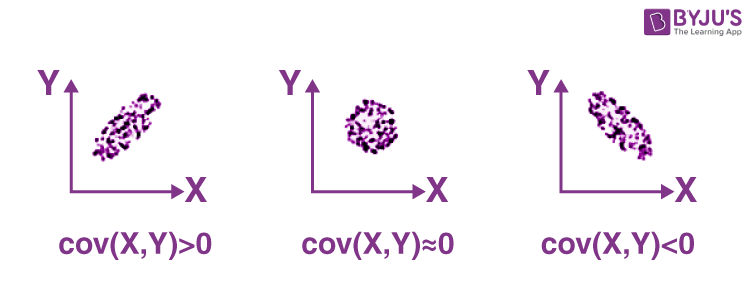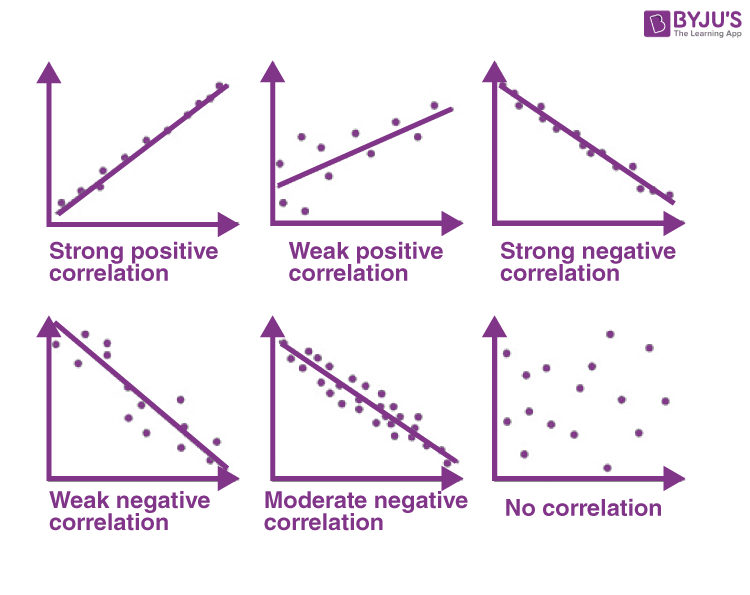Checkout JEE MAINS 2022 Question Paper Analysis : Checkout JEE MAINS 2022 Question Paper Analysis :

# Covariance

In statistics and probability theory, covariance deals with the joint variability of two random variables: x and y. Generally, it is treated as a statistical tool used to define the relationship between two variables. In this article, covariance meaning, formula, and its relation with correlation are given in detail.

## Covariance Meaning

Covariance is a measure of the relationship between two random variables and to what extent, they change together. Or we can say, in other words, it defines the changes between the two variables, such that change in one variable is equal to change in another variable. This is the property of a function of maintaining its form when the variables are linearly transformed. Covariance is measured in units, which are calculated by multiplying the units of the two variables.

## Types of Covariance

Covariance can have both positive and negative values. Based on this, it has two types:

1. Positive Covariance
2. Negative Covariance

### Positive Covariance

If the covariance for any two variables is positive, that means, both the variables move in the same direction. Here, the variables show similar behaviour. That means, if the values (greater or lesser) of one variable corresponds to the values of another variable, then they are said to be in positive covariance.

### Negative Covariance

If the covariance for any two variables is negative, that means, both the variables move in the opposite direction. It is the opposite case of positive covariance, where greater values of one variable correspond to lesser values of another variable and vice-versa.

## Covariance Formula

Covariance formula is a statistical formula, used to evaluate the relationship between two variables. It is one of the statistical measurements to know the relationship between the variance between the two variables. Let us say X and Y are any two variables, whose relationship has to be calculated. Thus the covariance of these two variables is denoted by Cov(X,Y). The formula is given below for both population covariance and sample covariance.Where,

• xi = data value of x
• yi = data value of y
• x̄ = mean of x
• ȳ = mean of y
• N = number of data values.

### Covariance of X and Y

Below figure shows the covariance of X and Y.If cov(X, Y) is greater than zero, then we can say that the covariance for any two variables is positive and both the variables move in the same direction.

If cov(X, Y) is less than zero, then we can say that the covariance for any two variables is negative and both the variables move in the opposite direction.

If cov(X, Y) is zero, then we can say that there is no relation between two variables.

### Correlation Coefficient Formula

We have already discussed covariance, which is the evaluation of changes between any two variables. Correlation estimates the depth of the relationship between variables. It is the estimated measure of covariance and is dimensionless. In other words, the correlation coefficient is a constant value always and does not have any units. The relationship between the correlation coefficient and covariance is given by;

 Correlation,ρ(X,Y) = Cov(X,Y)/σX σy

Where:

• ρ(X,Y) = correlation between the variables X and Y
• Cov(X,Y) = covariance between the variables X and Y
• σX = standard deviation of the X variable
• σY = standard deviation of the Y variable

Based on the value of correlation coefficient, we can estimate the type of correlation between the given two variables. Also, the graphical representation of correlation among two variables is given in the below figure.## Covariance and Correlation

Below table shows the comparison among covariance and correlation in brief.

 Covariance Correlation It is a measure to show the extent to which given two random variables change with respect to each other. It is a measure used to describe how strongly the given two random variables are related to each other. It is a measure of correlation. It is defined as the scaled form of covariance. The value of covariance lies between -∞ and +∞. The value of correlation lies between -1 and +1. It indicates the direction of the linear relationship between the given two variables. It measures the direction and strength of the linear relationship between the given two variables.

## Covariance and Variance

Covariance and variance both are the terms used in statistics. Variance is the measure of spread of data around its mean value but covariance measures the relation between two random variables. Learn Variance in statistics at BYJU’S.

## Covariance Example

Below example helps in better understanding of the covariance of among two variables.

Question:

Calculate the coefficient of covariance for the following data:

 X 2 8 18 20 28 30 Y 5 12 18 23 45 50

Solution:

Number of observations = 6

Mean of X = 17.67

Mean of Y = 25.5

Cov(X, Y) = (⅙) [(2 – 17.67)(5 – 25.5) + (8 – 17.67)(12 – 25.5) + (18 – 17.67)(18 – 25.5) + (20 – 17.67)(23 – 25.5) + (28 – 17.67)(45 – 25.5) + (30 – 17.67)(50 – 25.5)]

= 157.83

## Covariance Matrix

In statistics and probability theory, a square matrix provides the covariance between each pair of components (or elements) of a given random vector is called a covariance matrix. Any covariance matrix is symmetric and positive semi-definite. The principal diagonal or main diagonal (sometimes a primary diagonal) of this matrix contains variances. That means the covariance of each element with itself. A covariance matrix is also known as the auto-covariance matrix, variance matrix, dispersion matrix, or variance-covariance matrix.

## Frequently Asked Questions on Covariance

### What does Covariance mean?

Covariance, in statistics, is the measurement of relationships between two random variables.

### What is the difference between covariance and correlation?

Covariance describes the extent to which one variable is related to another whereas correlation states how strongly the given two random variables are related to each other.

### How to calculate the covariance?

To find the covariance, first find the mean of the set of data for two random variables.
Now find the difference between each value and the mean. Now add all the values and divide by the total number of values.

### Is covariance and variance same?

No, covariance and variance both are two different quantities in statistics.